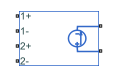# PVCCS2

Polynomial voltage-controlled current source with two controlling inputs

•Libraries:
Simscape / Electrical / Additional Components / SPICE Sources

## Description

The PVCCS2 (Two-Input Polynomial Voltage-Controlled Current Source) block represents a current source whose output current value is a polynomial function of the voltages across the pairs of controlling input ports. This equation describes the current through the source as a function of time:

`${I}_{out}={p}_{1}+{p}_{2}*{V}_{in1}+{p}_{3}*{V}_{in2}+{p}_{4}*{V}_{in1}^{2}+{p}_{5}{V}_{in1}*{V}_{in2}+{p}_{6}*{V}_{in2}^{2}+\dots$`

where:

• Vin1 is the voltage across the first pair of input ports.

• Vin2 is the voltage across the second pair of input ports.

• p is the Polynomial coefficients parameter value.

The block uses a small conductance internally to prevent numerical simulation issues. The conductance connects the output ports of the device and has a conductance GMIN:

• By default, GMIN matches the GMIN parameter of the Environment Parameters block, whose default value is `1e–12`.

• To change GMIN, add an Environment Parameters block to your model and set the GMIN parameter to the desired value.

## Ports

### Conserving

expand all

Electrical conserving port associated with the PVCCS2 positive voltage of the first controlling input.

Electrical conserving port associated with the PVCCS2 negative voltage of the first controlling input.

Electrical conserving port associated with the PVCCS2 positive voltage of the second controlling input.

Electrical conserving port associated with the PVCCS2 negative voltage of the second controlling input.

Electrical conserving port associated with the PVCCS2 positive output voltage.

Electrical conserving port associated with the PVCCS2 negative output voltage.

## Parameters

expand all

The polynomial coefficients that relate the input voltage to the output current, as described in the preceding section.

Specify if the current source includes a parallel conductance. The value of the parallel conductance, GMIN, depends on whether your model contains an Environment Parameters block.

This table shows the possible values of the parallel conductance, GMIN:

Values of parallel conductance, GMIN

Include parallel conductance, GMIN
`Yes``No`
Model contains Environment Parameters blockModel does not contain Environment Parameters blockParallel conductance, GMIN, is not included and it does not affect the current source.
GMIN is equal to the value of the GMIN parameter in the Environment Parameters block.GMIN = `1e-12` `Ohm`

## Version History

Introduced in R2009a